# Mimic IF Statements That Have Conditional Fields as Outputs

Introduction
Step-By-Step Instructions

# Introduction

Consider the following situation:

• You want to run an IF statement in a Computation, =IF([condition], [true output], [false output]). In the example below, this is =IF(A2>1000, "High Risk", A3).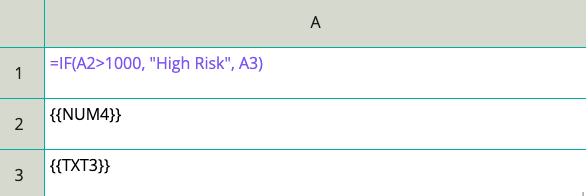Figure 1: IF statement in a Comp
• The IF statement's true or false output is a dynamic reference to the contents of a conditional field. In this example, the false output is A3, which contains {{TXT3}}, a Text field that the user will only answer if a particular condition is met.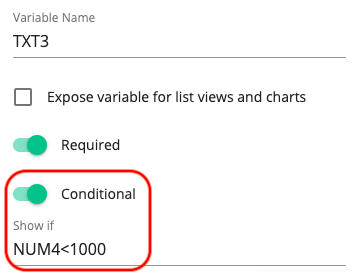Figure 2: A conditional text field. The IF statement in Figure 1 refers to this text field.

With this setup, if the TXT3 condition is not met and TXT3 never appears to the user, the IF statement will be invalid, as it will be referring to an output that does not exist. As a result, the cell with IF statement will not contain either the true or the false output when an Assessment is run.

This limitation with IF statements can be worked around with:

# Step-By-Step Instructions

Instead of using a single Comp with the IF statement described above: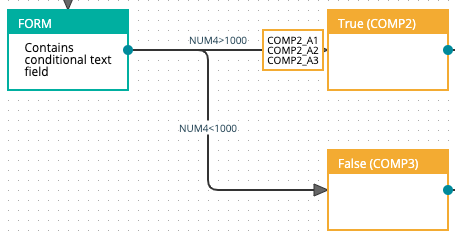Figure 3: Comp pages containing what would have been IF statement true and false outputs
2. In a cell of one of the Comp pages added in step 1., write the true output that would have been in the IF statement. In the other Comp, write the false output.
3.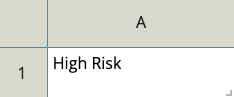Figure 4: True output written in COMP2_A1 (true output Comp) from FIgure 3. Output from IF statement described in Introduction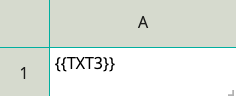Figure 5: False output written in COMP3_A1 (false output Comp). Output from IF statement described in Introduction
4. In the route connecting to the true output Comp, write the condition that would have been required for the IF statement to be true in the Route IF field. In the false output Comp route, write what would have been the false condition for the IF statement. This is pictured in Figure 3.
5. Connect both Comps from step 1. into a single Merge. In this Merge, add an Output Set with two Variable REFs, one containing the Comp cell with the true output and the other containing the false output cell. Note the LIST# variable name for this Merge Output Set.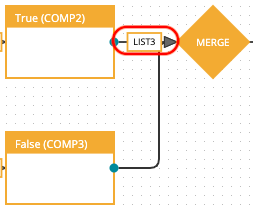Figure 6: True and false output Comps connected to a Merge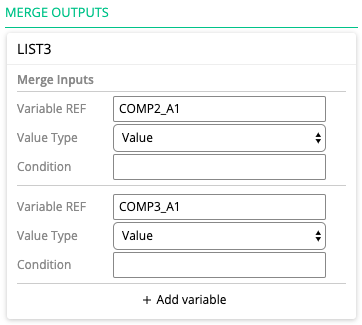Figure 7: True and false output Comp cells added in Variable REF fields of a single Merge Output Set
6. Add another Comp after the Merge. In a Comp cell, write ={{LIST#}}, LIST# being the Merge Output Set variable name.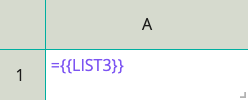Figure 8: ={{LIST}} trick applied to Merge Output Set in Figure 7. In this example, the ={{LIST}} trick is being recorded in COMP5_A1

The Comp cell in step 5. will contain what would have been the true or false output in the IF statement. This cell's variable name (COMP5_A1 in the example above) can be dynamically referenced as if it were a normal Comp IF statement output, and will not have the bullet point formatting that List variables usually have.